Question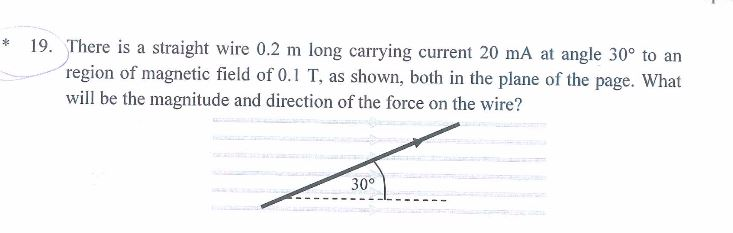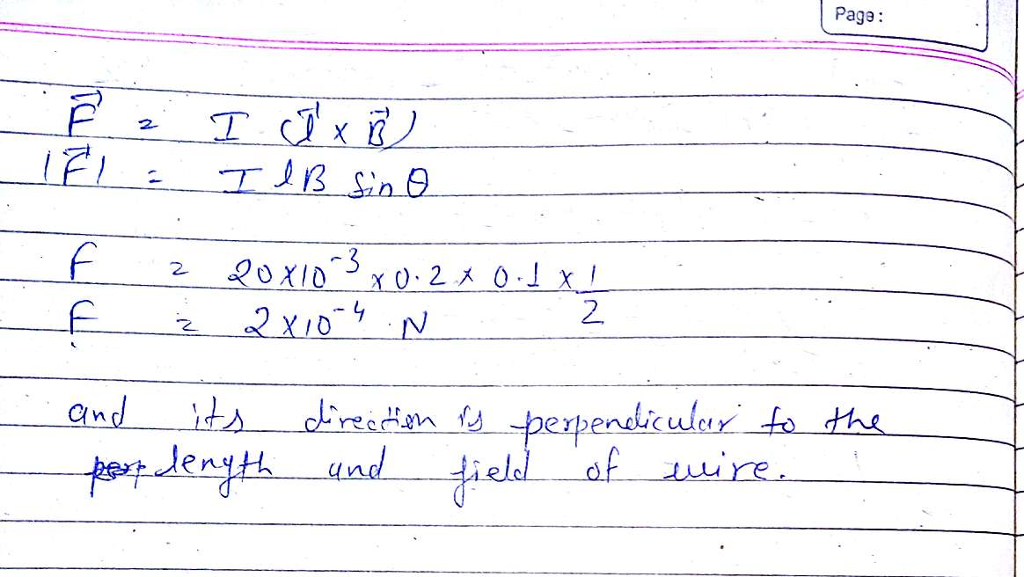#### Earn Coins

Coins can be redeemed for fabulous gifts.

Similar Homework Help Questions
• ### A long, straight wire is carrying a current of 5.0 A in the direction shown in...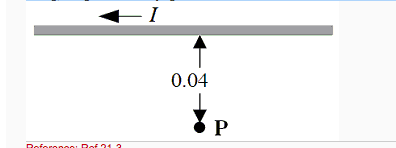A long, straight wire is carrying a current of 5.0 A in the direction shown in the figure. The point P is 0.040 m from the wire. What is the direction of the magnetic field at point P due to the current in the wire? Question 23 options: 1) toward the bottom of the page 2) to the left of the page 3) out of the plane of the page 4) into the plane of the page 5) to the...

• ### A uniform magnetic field of magnitude 25 points to the right. A 2.0-m-long wire, carrying 20...A uniform magnetic field of magnitude 25 points to the right. A 2.0-m-long wire, carrying 20 A, is placed at an angle of to the magnetic field, as shown in the figure. 30° What is the direction of the magnetic force on the wire? The magnetic force points O A to the right; OB out of the page: Cinto the page: OD to the left; E upward; OF downward

• ### 4. A long straight wire carrying a current is in the plane of a circular loop...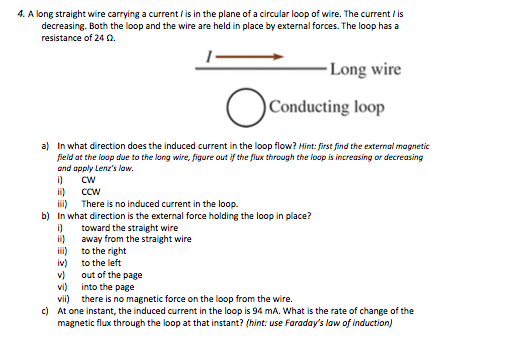4. A long straight wire carrying a current is in the plane of a circular loop of wire. The current is decreasing. Both the loop and the wire are held in place by external forces. The loop has a resistance of 24. -Long wire Conducting loop a) In what direction does the induced current in the loop flow? Mint: first find the external magnetic field at the loop due to the long wire, figure out if the flux through the...

• ### 11. A straight wire that is 0.60 m long is carrying a current of 2.0 A....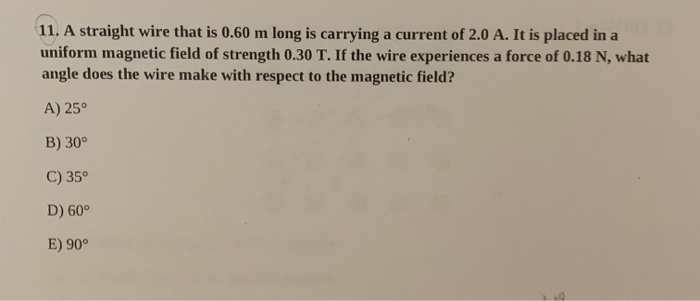11. A straight wire that is 0.60 m long is carrying a current of 2.0 A. It is placed in a uniform magnetic field of strength 0.30 T. If the wire experiences a force of 0.18 N, what angle does the wire make with respect to the magnetic field? A) 25° B) 30° C) 35° D) 60° E) 90°

• ### A flat rectangular wire loop is positioned next to a long straight current-carrying wire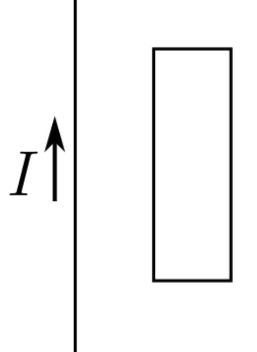Problem 6: A flat rectangular wire loop is positioned next to a long straight current-carrying wire. Both the loop and the wire are in the plane of the page, and the direction of the current is clearly indicated in the figure.Part (a) Which image best indicates the direction of the magnetic field due to the current in the long straight wire at a point inside the loop? Part (b) How does the magnitude of the magnetic field change as the perpendicular...

• ### A current-carrying wire moves toward a coil A long straight wire carrying current I is moving...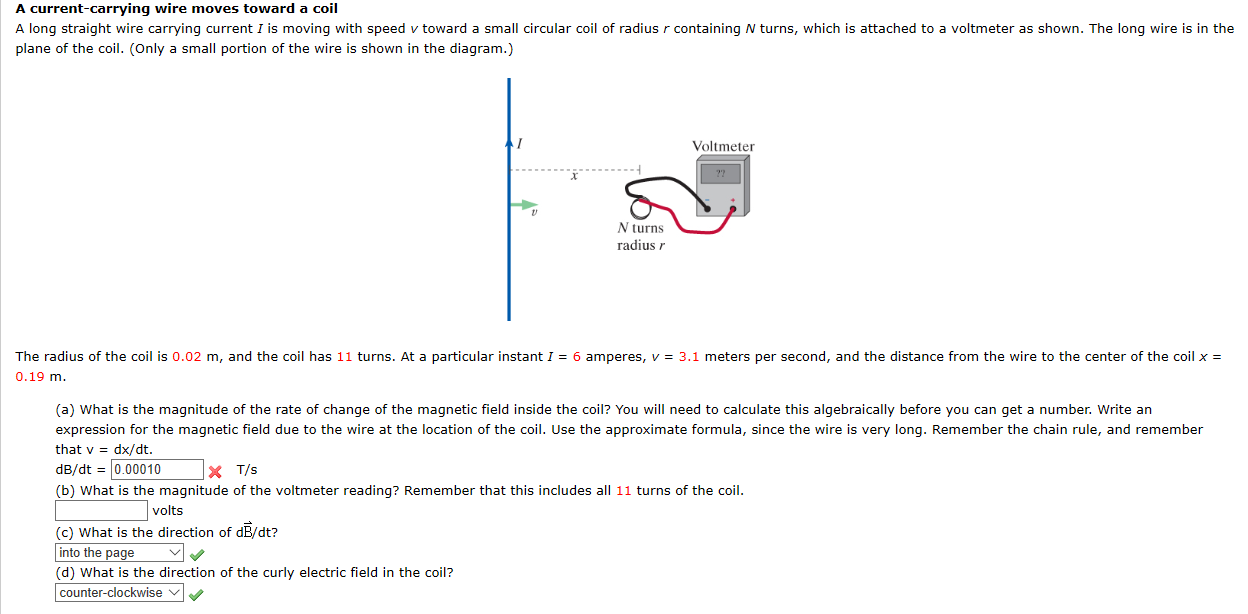A current-carrying wire moves toward a coil A long straight wire carrying current I is moving with speed v toward a small circular coil of radius r containing N turns, which is attached to a voltmeter as shown. The long wire is in the plane of the coil. (Only a small portion of the wire is shown in the diagram.) Voltmeter N turns radius r The radius of the coil is 0.02 m, and the coil has 11 turns. At...

• ### A long wire carrying a 6.00A current reverses direction by means of two right-angle bends, as...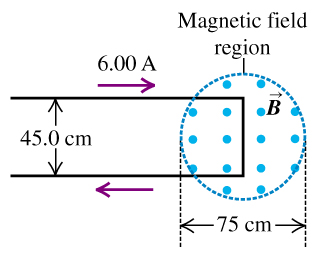A long wire carrying a 6.00A current reverses direction by means of two right-angle bends, as shown in the figure (Figure 1) . The part of the wire where the bend occurs is in a magnetic field of 0.666T confined to the circular region of diameter 75cm, as shown. A) Find the direction of the net force that the magnetic field exerts on this wire. B) Find the magnitude of the net force that the magnetic field exerts on this...

• ### A long wire carrying 4.50 A of current makes two 90 degree bends, as shown in....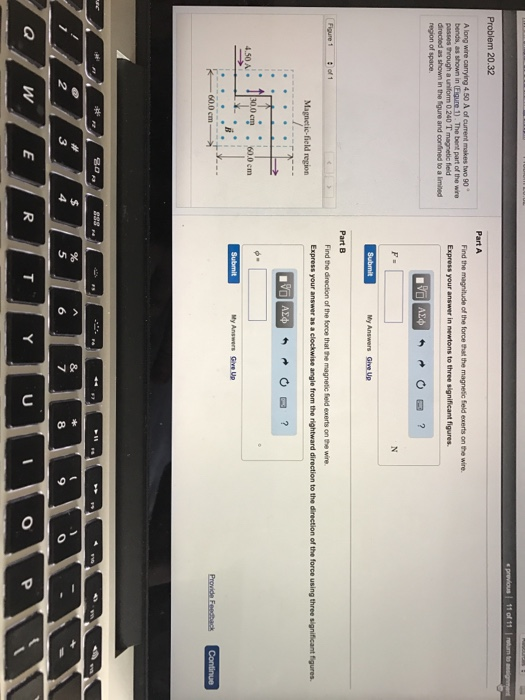A long wire carrying 4.50 A of current makes two 90 degree bends, as shown in. The best part of the wire passes through a uniform 0.240 t magnetic field directed as shown in the figure and confined to a limited of space. Part A Find the magnitude of the force that the magnetic field exerts on the wire Part B Find the direction of the force that the magnetic field exerts on the wire.

• ### A straight wire carries a current of 10 A at an angle of 30* with respect...

A straight wire carries a current of 10 A at an angle of 30* with respect to the direction of a uniform 0.30 T magnetic field. Find the angle the magnitude of the magnetic force on a 0.50 m length of the wire.

• ### A long wire is bent to form a 90 degree angle using a circular arc with...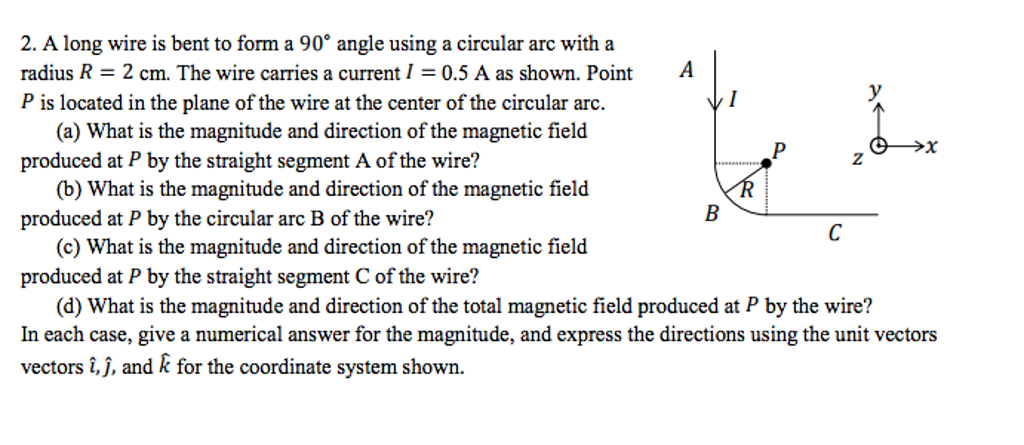A long wire is bent to form a 90 degree angle using a circular arc with a radius r=2cm. The wire carries a current I=0.5A as shown. Point P is located in the plane of the wire at the center of the circular arc. 2. A long wire is bent to form a 90° angle using a circular arc with a radius R 2 cm. The wire carries a current I = 0.5 A as shown. Point P is located...